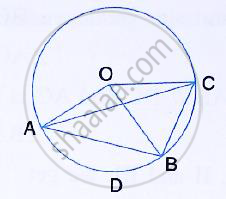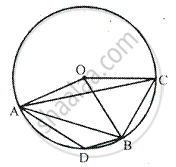Share

# In the Figure, O is the Centre of the Circle and the Length of Arc Ab is Twice the Length of Arc Bc. If Angle Aob = 108°, Find: - Mathematics

Course

#### Question

In the figure, O is the centre of the circle and the length of arc AB is twice the length of arc BC. If angle AOB = 108°, find : ∠CAB#### SolutionArc B = 2 arc BC and ∠AOB = 180°

∴ ∠BOC = 1 ∠AOB
= 1/2 xx108°
= 54°

Now, Arc BC subtends ∠BOC at the centre and

∠CAB at the remaining part of the circle.

∴ ∠CAB = 1/2 ∠BOC
= 1/2xx  54°
= 27°

Is there an error in this question or solution?